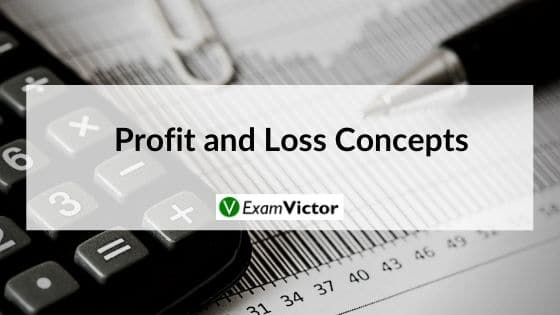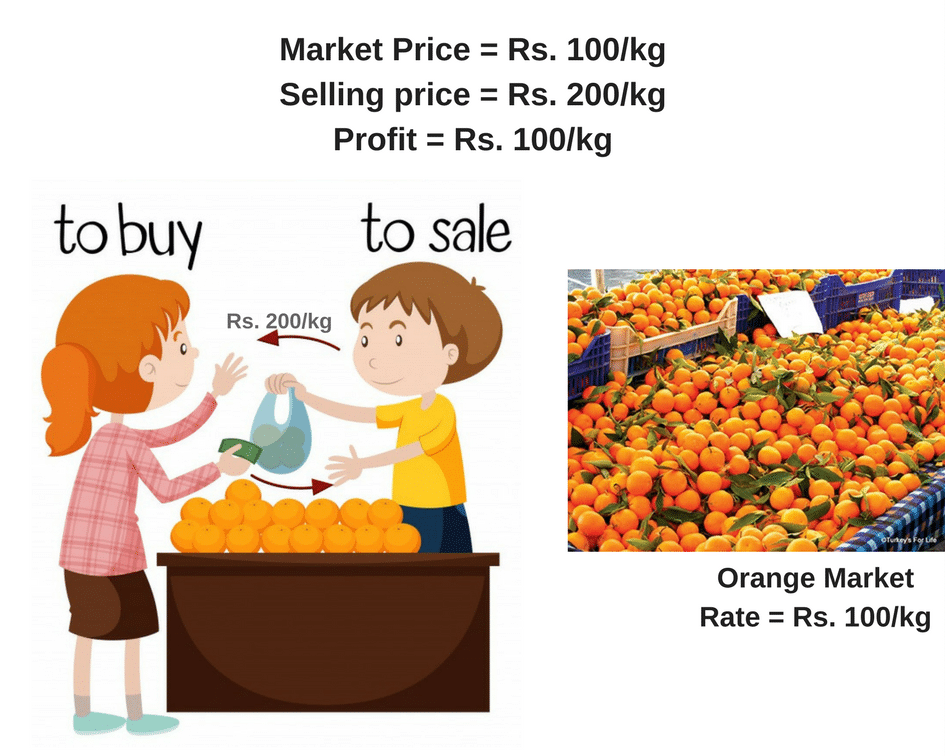# Profit and Loss ConceptsLast Updated on Nov 9, 2020

We have been studying concepts of profit and loss since class 6th. Till then we have studied it just to score good marks in mathematics, but have you observed it’s presence in our day-to-day life. It is present in our daily transactions from buying grocery to buying company stocks. Everything we pay for includes profit and loss. From a shop to the big MNC’s has been running with a single intention of earning profit. Whenever a seller want to sell out something, he is always looking to earn some profit.

Every businessman’s motive is to earn profit, but in the process they also incur some loss. Profit and loss are part and parcel on any business. Finally at the end of the financial year every businessman has earned either profit or loss. Before venturing any business, it is very necessary to build it’s profit model and hence this topic is so significant and included in the CAT exam. Le’s begin with the basics of profit and loss.

Let’s consider an example to understand it more better,

Suppose you went to shop and bought 2 t-shirt for Rs. 500 each. You come to your hostel after shopping and your friend asks you to have a look at the thing you have shopped for. He liked one of your t-shirt and asked it’s price. You lie to him and said that you bought it for Rs. 700 each and then he ask you to give him one of the t-shirt for Rs. 700. In the given case, you lied to your friend and earned Rs. 200 more than what you paid for the t-shirt, this is how you made the profit.

In the given example Rs. 500 is the cost price and Rs. 700 is the selling price for you.
Profit – selling price – cost price = 700-500 = 200

From the given example we have understood that when selling price > cost price means profit, otherwise loss.

One more example from day-to- day life is shown in the image below:### Profit and Loss Definitions

Cost Price: The price (amount) paid to purchase a product or the cost incurred in manufacturing a product is known as the cost price (CP) of that product.

#### Types of cost

1. Fixed cost: It is a type of cost which is fixed under all conditions and does not vary according to the number of units produced.
2. Variable cost: Variable cost is a type of cost which varies according to the number of units. This is quite easy to understand.
3. Semi-variable cost: As the name suggests, these costs are the ones that are fixed in part and variable in part. Effectively, this is the case that we see most often. Imagine the scenario in a factory. There is a capital cost, which remains the same under all conditions (fixed cost) and a variable cost of the product, which in turn depends upon various factors.

Selling Price: The price at which a product is sold is called the selling price (SP) of the product.
Marked Price: Do shopkeepers put up a price on the label that they wish to sell on or they put up an inflated price? If you think closely, the majority of the shopkeepers’ mark-up their products, in anticipation of the discounts they would have to offer. This is a clever way of operating. Mark-up the price in advance, offer a discount and make the customer feel happy, and then sell the product. Pretty effective, eh?

List Price: List price or the tag price is the price that is printed on the tag of the article. For all practical purposes, we assume it to be same as the marked-price.

Margin: The profit percentage on selling price is known as MARGIN.

Example 1: Savita sell an article at a discount of 80% and get a profit of 60% on that article to calculate the mark up over the cost price?

Solution:
Let us put the above definitions in use.
Let us assume that Cost Price = Rs 100.
So, Selling Price = Rs 160.
Now, after giving a discount of 80% over MP, Rs 160 is the SP.
Let the Marked Price: MP
SP = 20% of MP
160 = 20% of MP
MP = 800
% Mark Up = (700/100) × 100 = 700 %.

Things to remember in this question:
Mark-Up over Cost Price = Marked Price – Cost Price = M
% Mark Up Cost Price = [{M/CP} x 100]

Mark-Up over Selling Price = Marked Price – Selling Price = M
% Mark Up Selling Price = [{M/SP} x 100]

Note: Focus closely on what is being asked. Remember Profit and Loss is nothing else but an application of Percentages and basic calculation skills.

Let’s consider another example.

Example 2: Ram bought a camera and paid 20% less than its original price. He sold it at a 40% profit on the price he had paid. The per­centage of profit earned by Ram on the original price was
(1) 22%
(2) 32%
(3) 12%
(4) 15%

Explanation:
CP= Cost Price, SP= Selling Price
Let the original price be Rs. p.

CP = (80/100)p=Rs. 4p/5
SP = (4P/5)x(140/100) = Rs. 28p/25

Gain on original price=sp-cp = 28p/25 – p = 3p/25

Therefore Gain % = (Gain/ Original Price ) x 100

Gain Percentage = {Gain /(Original Price ) x 100}

Gain Percentage = (3p/25p) x 100 = 12%

### Summary

In this blog post, we have explained the basics of Profit and Loss taking some easy examples and we have solved them explaining them well. We hope you liked our post. Share your views in the comment section given below and for much such post click here.